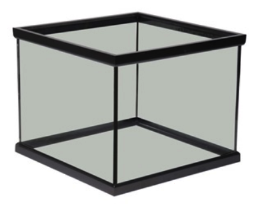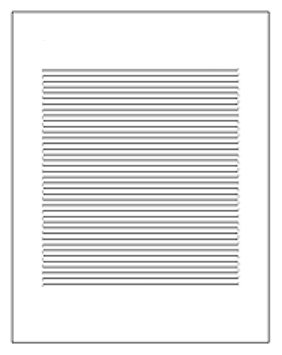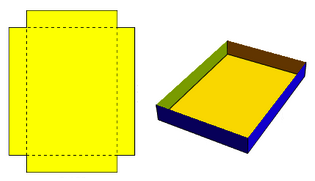Optimization

Armed with the Extreme Value Theorem, Fermat's Theorem, the ability to find critical values for a function, and the first and second derivative tests, We are now able to quickly find points where a function obtains a maximum or minimum value.

Consider the practical applications that we can now address!

If we can find functions to model revenue, cost, distances, concentrations, spread of a disease, flow, or any of a number of other things whose maximizing or minimizing might be important -- we can now use calculus to find out exactly what the values of those maximums or minimums are and where they occur!

The class of problems where one seeks to find useful extrema relative to some practical application are known as optimization problems. The following provides some examples of these:

ExampleA rectangular, open-topped, square-bottomed aquarium is to hold 1 cubic meter of water. Given that the material for the bottom costs twice as much per square meter as that for the sides, find the dimensions of the least expensive aquarium.

Solution:

Suppose the square base has side length $x$ meters and the height is $h$ meters. The cost is then clearly proportional to $C = 2x^2 + 4xh$. Thus, minimizing $C$ will minimize the cost of the aquarium.

Note that $C$ is a function of two variables, $x$ and $h$. Indeed, we might even write $C(x,h) = 2x^2 + 4xh$ to emphasize this point.

Unfortunately, we have not yet seen in how to find maxima or minima of functions of two variables. Without any additional information, we would have to wait until the calculus of functions of two or more variables is addressed in a later course (which for reasons that may now make some sense, is called multivariable calculus) before we can solve such problems.

Fortunately however for us, in this particular situation there is some additional information in the problem -- information that we can exploit to reduce the function to be minimized down to a function of just a single variable!

Note that the given volume tells us $x^2h = 1$. We can use this equation to find an expression for $h$ in terms of $x$, which we can then use to replace the $h$ in cost equation. This will then give us the cost in terms of only the single variable $x$.

Doing this, we find $\displaystyle{h = \frac{1}{x^2}}$. Then, upon substitution we find cost as a function of only $x$:

$$C(x) = 2x^2 + 4x\left(\frac{1}{x^2}\right) = 2x^2 + \frac{4}{x}$$

Now finally, the tricks we know for finding maxima and minima come to bear -- we search for critical points, endpoints, etc., where these extrema can happen.

That said, we need to be careful. How would we interpret things if $C(x)$ ends up having an absolute minimum at some negative value of $x$?

While we can evaluate $C(x)$ for negative values of $x$, the dimensions of the related aquarium don't make any sense when $x$ is negative. Think about it, $x$ is a side length for the square base -- it can't be negative!

Such things often happen in optimization problems -- the domain of the function to maximized or minimized is not only impacted by what is algebraically computable, but also by physical constraints of what the variables represent. For example, as we have just seen, if the variables represent any kind of dimensions (e.g., lengths, areas, volumes), they can't be negative. Indeed, zero can be a problem too -- consider physically building a box with dimensions $2$ ft $\times$ $3$ ft $\times$ $0$ ft!

In this problem, we certainly thus need $x \gt 0$. However, we should also check to make sure $h$ is reasonable too, as it is a height and must also be positive. Fortunately in this case, we note $h = 1/x^2$ which is always positive anyways. So here, the physical nature of $h$ doesn't add any additional restrictions on $x$ -- but in other contexts it might have.

Consequently, we seek to minimize $\displaystyle{C(x) = 2x^2 + \frac{4}{x}}$ on $(0,\infty)$.

At this point, the calculations are routine. We first find that $C(x)$ has derivative $$C'(x) = 4x - \frac{4}{x^2} = \frac{4(x^3-1)}{x^2}$$ which is undefined at $x=0$ and zero at $x=1$.

A sign analysis of the first derivative in our domain $(0,\infty)$ reveals

Thus, on $(0,\infty)$ we have an absolute minimum at $x=1$ ft.

With $x=1$, it must also then be the case that $h = 1/1^2 = 1$. Now we have the dimensions of the least expensive aquarium: $1$ m $\times$ $1$ m $\times$ $1$ m.

ExampleA page is to contain 30 in2 of print. The margins at the top and the bottom of the page are each 2 in wide. The margins on each side of the page are only 1 in wide. Find the dimensions of the page so that the least amount of paper is used.

Solution:

Suppose the text is $x$ inches wide and $y$ inches high.

We seek to minimize the area of the page $A = (x+2)(y+4)$. We currently have $A$ as a function of $2$ variables, whereas we would prefer to have $A$ as a function of only a single variable -- so that we could use our understanding of how to find maxima and minima of such functions with calculus.

We need some additional information -- some relationship between $x$ and $y$ that we can exploit to this end.

Fortunately, we know the area of the text on the page is 30 in2. Thus, $xy = 30$ and consequently $\displaystyle{y = \frac{30}{x}}$. Having solved for $y$ in terms of $x$, we can now replace the $y$ in the expression for $A$ to get $A$ as a function of only one variable.

$$A(x) = (x+2)\left(\frac{30}{x} + 4\right)$$

Expanding the product above tells us

$$A(x) = \frac{60}{x} + 4x + 38$$ with derivative given by $$A'(x) = -\frac{60}{x^2} + 4 = \frac{4(x^2-15)}{x^2}$$

which is zero when $x = \pm \sqrt{15}$ and undefined at $x=0$.

Of course, we can ignore the negative and zero values of $x$ as it makes no sense for the width of the text to be either of these. We should also note that the height should similarly not be zero or negative, so $y+4 \gt 0$. This in turn tells us to only focus on those $x$ values where $\frac{30}{x} \gt 0$. Fortunately, this doesn't add any restrictions on $x$ as it is equivalent to $x \gt 0$.

So to find $x$ that minimizes $A(x)$ on $(0,\infty)$, we need only do a sign analysis of $A'(x)$ on this domain:

to discover an absolute minimum for $A(x)$ at $x=\sqrt{15}$.

Finally, compute $\displaystyle{y = \frac{30}{\sqrt{15}} = 2\sqrt{15}}$ to determine the paper dimensions: $(\sqrt{15} + 2) \times (2\sqrt{15} + 4)$

ExampleRectangular boxes are formed by cutting squares of equal sizes from the corners of rectangular sheets of cardboard and by folding up the remaining material. Find the dimensions of the box of largest volume which can be formed from a 5 ft $\times$ 8 ft sheet of cardboard.

Solution:

Suppose the squares that are removed from the corners have side length $x$. Then we seek to maximize the volume of the box formed, which is given by

$$V(x) = x (8-2x)(5-2x) = 40x - 26x^2 + 4x^3$$

$V$ is nicely already a function of one variable, so we will not have to play the games we did in the previous examples to make that happen.

We do want to remember that since $x$ is a length, it must be positive -- but there is also another restriction on $x$. Notice that the squares we remove can not be larger than half the shortest dimension of our cardboard. That is to say, if we cut out squares where $x\ge\frac{5}{2}$, there will be no side to turn up on the shorter sides of the cardboard! Thus, we seek to maximize $V(x)$ on $(0,\frac{5}{2})$.

Finding the derivative

$$V'(x) = 40-52x+12x^2 = 4(3x^2-13x+10) = 4(x-1)(3x-10)$$

leads us to consider $x=\frac{10}{3}$ and $x=1$, although $\frac{10}{3}$ lies outside $(0,\frac{5}{2})$ and should thus be discarded.

A sign analysis of $V'(x)$ confirms that the maximum on the interval in question occurs at $x=1$.

Finding $x=1$, the dimensions of the box are then $1$ ft $\times$ $6$ ft $\times$ $3$ ft.